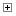##Classes

Defined in: qdt.js.

Class Summary
Constructor Attributes Constructor Name and Description

Field Summary
Field Attributes Field Name and Description
<private>
Internal _query method that lacks adjustment of the parameter.

In a subdivided quad tree this represents the top right subtree.

In a subdivided quad tree this represents the top left subtree.

Point storage.

In a subdivided quad tree this represents the bottom right subtree.

In a subdivided quad tree this represents the bottom left subtree.
Method Summary
Method Attributes Method Name and Description

contains(x, y)
Checks if the given coordinates are inside the quad tree.

insert(p)
Insert a new point into this quad tree.

Class Detail
Parameters:
{Array} bbox
Bounding box of the new quad (sub)tree.

Field Detail
Internal _query method that lacks adjustment of the parameter.

northEast
In a subdivided quad tree this represents the top right subtree.

northWest
In a subdivided quad tree this represents the top left subtree.

{Array} points
Point storage.

southEast
In a subdivided quad tree this represents the bottom right subtree.

southWest
In a subdivided quad tree this represents the bottom left subtree.
Method Detail
{Boolean} contains(x, y)
Checks if the given coordinates are inside the quad tree.
Parameters:
{Number} x
{Number} y
Returns:
{Boolean}

{Boolean} insert(p)
Insert a new point into this quad tree.
Parameters:
p
Returns:
{Boolean}

subdivide()